# RS Aggarwal Class 7 Solutions Chapter 11 Profit and Loss Ex 11B

In this chapter, we provide RS Aggarwal Solutions for Class 7 Chapter 11 Profit and Loss Ex 11B for English medium students, Which will very helpful for every student in their exams. Students can download the latest RS Aggarwal Solutions for Class 7 Chapter 11 Profit and Loss Ex 11B Maths pdf, free RS Aggarwal Solutions Class 7 Chapter 11 Profit and Loss Ex 11B Maths book pdf download. Now you will get step by step solution to each question.

### RS Aggarwal Solutions for Class 7 Chapter 11 Profit and Loss Ex 11B Download PDF

Question 1.
Solution:
(b)
C.P. = Rs. 80
S.P. = Rs. 100
Gain = S.P. – C.P. = Rs. 100 – 80 = Rs. 20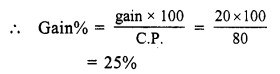Question 2.
Solution:
(a)
C.P. = Rs. 120
S.P. = Rs. 105
Loss = C.P. – S.P. = Rs. 120 – 105 = Rs. 15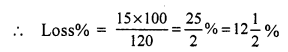Question 3.
Solution:
(b)
S.P. = Rs. 100
Gain = Rs. 20
C.P. = S.P. – gain = Rs. 100 – 20 = Rs. 80Question 4.
Solution:
(a)
S.P. = Rs. 198
Gain = 10%Question 5.
Solution:
(a)
First S.P. of jug = Rs. 144
Loss = 17 of C.P.
Let C.P. = xQuestion 6.
Solution:
(d)
First S.P. of a pen = Rs. 48
Loss = 20%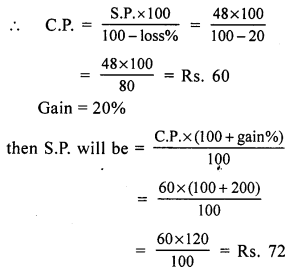Question 7.
Solution:
(a)
C.P. of 12 pencils = S.P. of 15 pencils = Re 1 (suppose)Question 8.
Solution:
(d)
Let CP of 4 toffees = SP of 3 toffee = Rs. 12
(LCM of 4, 3 = 12)
CP of 1 toffee = Rs. 124 = Rs. 3
SP of 1 toffee = Rs. 123 = 4
Gain = Rs. 4 – 3 = Re 1Question 9.
Solution:
(c) SP of an article = Rs. 144
Loss% = 10%Question 10.
Solution:
(a)
CP of 6 lemons = Re 1
CP of 1 lemon = Re 16
SP of 4 lemons = Re 1
SP of 1 lemon = Re 14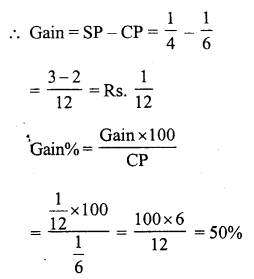Question 11.
Solution:
(d)
SP of chair = Rs. 720
Gain% = 20%Question 12.
Solution:
(c)
SP of stool = Rs. 630
Loss% = 10%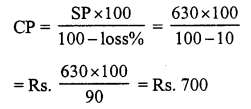All Chapter RS Aggarwal Solutions For Class 7 Maths

—————————————————————————–

All Subject NCERT Exemplar Problems Solutions For Class 7

All Subject NCERT Solutions For Class 7

*************************************************

I think you got complete solutions for this chapter. If You have any queries regarding this chapter, please comment on the below section our subject teacher will answer you. We tried our best to give complete solutions so you got good marks in your exam.

If these solutions have helped you, you can also share rsaggarwalsolutions.in to your friends.